Note: The other languages of the website are Google-translated. Back to English

## How to generate all combinations of 3 or multiple columns in Excel?

Supposing, I have 3 columns of data, now, I want to generate or list all combinations of the data in these 3 columns as below screenshot shown. Do you have any good methods for solving this task in Excel?Generate all combinations based on 3 columns of data by using a formula

Generate all combinations based on 3 or multiple columns of data by using VBA code

Generate all combinations based on 3 or multiple columns of data by using an awesome feature

#### Generate all combinations based on 3 columns of data by using a formula

The following long formula can help to list all combinations of 3 columns, please do as this:

1. Please, click a cell where to output the result, and then copy and paste the below formula into it:

=IFERROR(INDEX(\$A\$2:\$A\$4,INT((ROW(1:1)-1)/((COUNTA(\$B\$2:\$B\$6)*(COUNTA(\$C\$2:\$C\$5)))))+1)&"-"&INDEX(\$B\$2:\$B\$6,MOD(INT((ROW(1:1)-1)/COUNTA(\$C\$2:\$C\$5)),COUNTA(\$B\$2:\$B\$6))+1)&"-"&INDEX(\$C\$2:\$C\$5,MOD((ROW(1:1)-1),COUNTA(\$C\$2:\$C\$5))+1),"")

Note: In this formula: A2:A4, B2:B6, C2:C5 are the data ranges that you want to use.

2. Then, drag the fill handle down to the cells until blank cells are displayed, that means, all combinations of the 3 columns have been listed, see screenshot: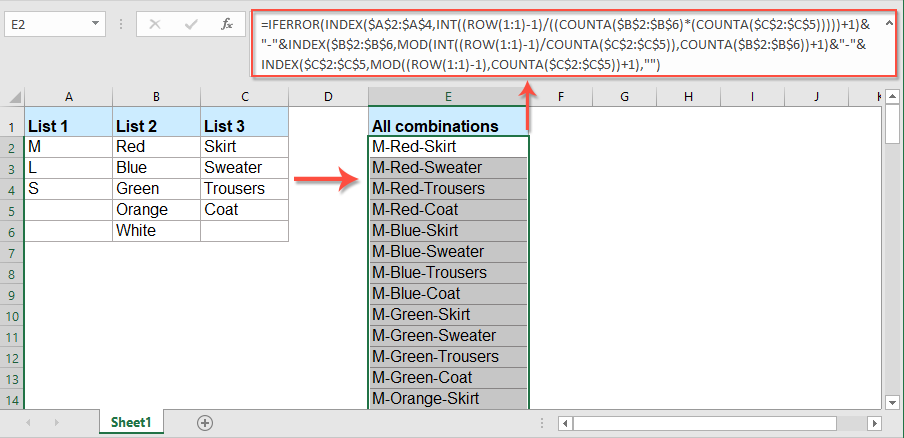#### Generate all combinations based on 3 or multiple columns of data by using VBA code

The above long formula is somewhat difficult for using, if there are multiple columns data need to use, it will be troublesome for modifying. Here, I will introduce a VBA code to deal with it quickly.

1. Hold down the ALT + F11 keys to open the Microsoft Visual Basic for Applications window.

2. Click Insert > Module, and paste the following code in the Module Window.

VBA code: Generate all combinations of 3 or multiple columns

``````Sub ListAllCombinations()
'Updateby Extendoffice
Dim xDRg1, xDRg2, xDRg3 As Range
Dim xRg  As Range
Dim xStr As String
Dim xFN1, xFN2, xFN3 As Integer
Dim xSV1, xSV2, xSV3 As String
Set xDRg1 = Range("A2:A4")  'First column data
Set xDRg2 = Range("B2:B6")  'Second column data
Set xDRg3 = Range("C2:C5")  'Third column data
xStr = "-"   'Separator
Set xRg = Range("E2")  'Output cell
For xFN1 = 1 To xDRg1.Count
xSV1 = xDRg1.Item(xFN1).Text
For xFN2 = 1 To xDRg2.Count
xSV2 = xDRg2.Item(xFN2).Text
For xFN3 = 1 To xDRg3.Count
xSV3 = xDRg3.Item(xFN3).Text
xRg.Value = xSV1 & xStr & xSV2 & xStr & xSV3
Set xRg = xRg.Offset(1, 0)
Next
Next
Next
End Sub
``````

Note: In the above code, A2:A4, B2:B6, C2:C5 are the data range that you want to use, E2 is the output cell that you want to locate the results. If you want to get all combinations of more columns, please change and add other parameters to the code as your need.

3. Then, press F5 key to run this code, and all combinations of the 3 or multiple columns will be generated at once, see screenshot:#### Generate all combinations based on 3 or multiple columns of data by using an awesome feature

If you have Kutools for Excel, with its powerful List All Combinations feature, you can list all combinations of multiple columns quickly and easily.

Tips:To apply this List All Combinations feature, firstly, you should download the Kutools for Excel, and then apply the feature quickly and easily.

After installing Kutools for Excel, please do as this:

1. Click Kutools > Insert > List All Combinations, see screenshot: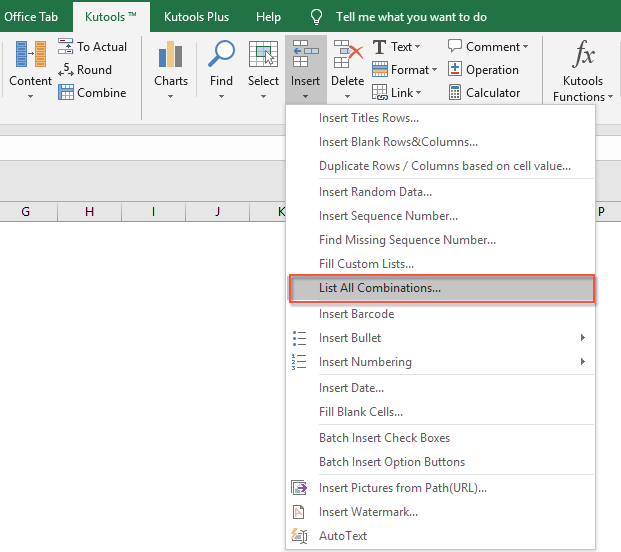2. In the List All Combinations dialog box, specify the columns data and separators for listing the combinations as following screenshot shown: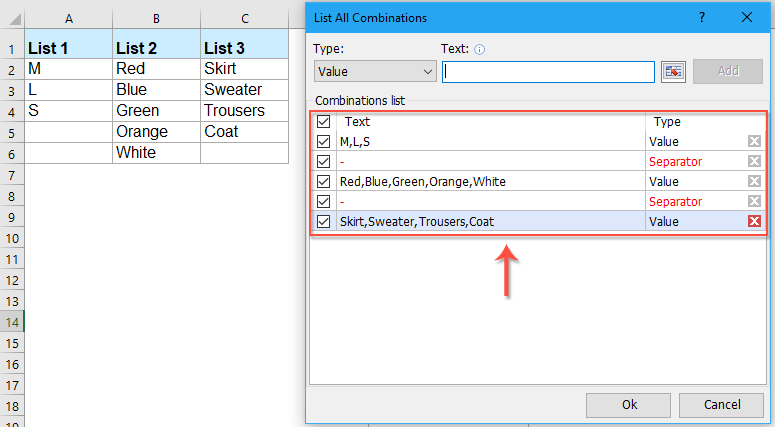3. After setting the data and separator, then, click Ok button, in the next prompt box, select a cell to locate the result, see screenshot: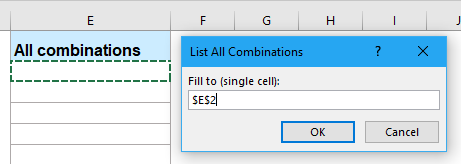4. And then, click OK button, all combinations have been generated immediately as below screenshot shown:## The Best Office Productivity Tools

### Kutools for Excel Solves Most of Your Problems, and Increases Your Productivity by 80%

• Super Formula Bar (easily edit multiple lines of text and formula); Reading Layout (easily read and edit large numbers of cells); Paste to Filtered Range...
• Merge Cells/Rows/Columns and Keeping Data; Split Cells Content; Combine Duplicate Rows and Sum/Average... Prevent Duplicate Cells; Compare Ranges...
• Select Duplicate or Unique Rows; Select Blank Rows (all cells are empty); Super Find and Fuzzy Find in Many Workbooks; Random Select...
• Exact Copy Multiple Cells without changing formula reference; Auto Create References to Multiple Sheets; Insert Bullets, Check Boxes and more...
• Favorite and Quickly Insert Formulas, Ranges, Charts and Pictures; Encrypt Cells with password; Create Mailing List and send emails...
• Extract Text, Add Text, Remove by Position, Remove Space; Create and Print Paging Subtotals; Convert Between Cells Content and Comments...
• Super Filter (save and apply filter schemes to other sheets); Advanced Sort by month/week/day, frequency and more; Special Filter by bold, italic...
• Combine Workbooks and WorkSheets; Merge Tables based on key columns; Split Data into Multiple Sheets; Batch Convert xls, xlsx and PDF...
• Pivot Table Grouping by week number, day of week and more... Show Unlocked, Locked Cells by different colors; Highlight Cells That Have Formula/Name...### Office Tab - brings tabbed interface to Office, and make your work much easier

• Enable tabbed editing and reading in Word, Excel, PowerPoint, Publisher, Access, Visio and Project.
• Open and create multiple documents in new tabs of the same window, rather than in new windows.
• Increases your productivity by 50%, and reduces hundreds of mouse clicks for you every day!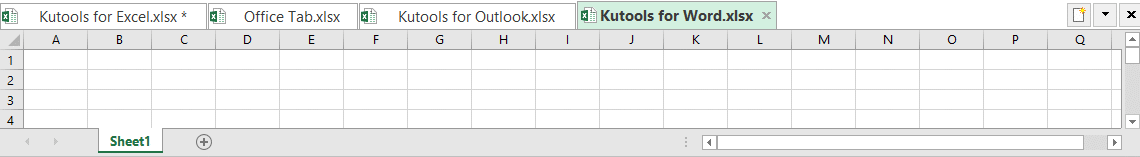No ratings yet. Be the first to rate!
This comment was minimized by the moderator on the site
To generate any random combination we can use
=INDEX(\$A\$2:\$A\$4,RANDBETWEEN(1,3))&"-"&INDEX(\$B\$2:\$B\$6,RANDBETWEEN(1,5))&"-"&INDEX(\$C\$2:\$C\$5,RANDBETWEEN(1,4))
This comment was minimized by the moderator on the site
Thanks a lot for sharing this post. Thanks a lot to @Balaji for his/her formula
This comment was minimized by the moderator on the site
I CANNOT THANK YOU ENOUGH! SAVED ME SO MUCH TIME!
This comment was minimized by the moderator on the site
HOw to do below formula for 5 columns? Trying to figure out but its giving error
=IFERROR(INDEX(\$A\$2:\$A\$4,INT((ROW(1:1)-1)/((COUNTA(\$B\$2:\$B\$6)*(COUNTA(\$C\$2:\$C\$5)))))+1)&"-"&INDEX(\$B\$2:\$B\$6,MOD(INT((ROW(1:1)-1)/COUNTA(\$C\$2:\$C\$5)),COUNTA(\$B\$2:\$B\$6))+1)&"-"&INDEX(\$C\$2:\$C\$5,MOD((ROW(1:1)-1),COUNTA(\$C\$2:\$C\$5))+1),"")
This comment was minimized by the moderator on the site
Hello, Nikhil,For getting all combinations from 5 columns, may be the below VBA code can help you, please change the cell references to your data.
<div data-tag="code">Sub ListAllCombinations()
'Updateby Extendoffice
Dim xDRg1, xDRg2, xDRg3, xDRg4, xDRg5 As Range
Dim xRg As Range
Dim xStr As String
Dim xFN1, xFN2, xFN3, xFN4, xFN5 As Integer
Dim xSV1, xSV2, xSV3, xSV4, xSV5 As String
Set xDRg1 = Range("A2:A7") 'First column data
Set xDRg2 = Range("B2:B7") 'Second column data
Set xDRg3 = Range("C2:C7") 'Third column data
Set xDRg4 = Range("D2:D7") 'Fourth column data
Set xDRg5 = Range("E2:E7") 'Fifth column data
xStr = "-" 'Separator
Set xRg = Range("H2") 'Output cell
For xFN1 = 1 To xDRg1.Count
xSV1 = xDRg1.Item(xFN1).Text
For xFN2 = 1 To xDRg2.Count
xSV2 = xDRg2.Item(xFN2).Text
For xFN3 = 1 To xDRg3.Count
xSV3 = xDRg3.Item(xFN3).Text
For xFN4 = 1 To xDRg4.Count
xSV4 = xDRg4.Item(xFN4).Text
For xFN5 = 1 To xDRg5.Count
xSV5 = xDRg5.Item(xFN5).Text
xRg.Value = xSV1 & xStr & xSV2 & xStr & xSV3 & xStr & xSV4 & xStr & xSV5
Set xRg = xRg.Offset(1, 0)
Next
Next
Next
Next
Next
This comment was minimized by the moderator on the site
Hello skyyang, I changed the code for 7 rolls, but seeing Excel has only 1,048,576 rows the VBA code can't give all the combinations.Do you have an idea how I can continue on other columns? I think this code - Set xRg = xRg.Offset(1, 0)needed to change
This comment was minimized by the moderator on the site
Hello skyyang, I changed the code for 7 rolls, but seeing Excel has only 1,048,576 rows the VBA code can't give all the combinations.
Do you have an idea how I can continue on other columns?
I think this code - Set xRg = xRg.Offset(1, 0)
needed to change
This comment was minimized by the moderator on the site
=IFERROR(INDEX(\$A\$2:\$A\$5,INT((ROW(1:1)-1)/((COUNTA(\$B\$2:\$B\$3)*(COUNTA(\$C\$2:\$C\$3)* (COUNTA(\$D\$2:\$D\$4)* (COUNTA(\$E\$2:\$E\$6)* (COUNTA(\$H\$2:\$H\$6)* (COUNTA(\$G\$2:\$G\$6)* (COUNTA(\$H\$2:\$H\$6))))))))))+1)&"-"&INDEX(\$B\$2:\$B\$3,MOD(INT((ROW(1:1)-1)/COUNTA(\$C\$2:\$C\$3)),COUNTA(\$B\$2:\$B\$3))+1)&"-" &INDEX(\$C\$2:\$C\$3,MOD(INT((ROW(1:1)-1)/COUNTA(\$D\$2:\$D\$4)),COUNTA(\$C\$2:\$C\$3))+1)&"-" &INDEX(\$D\$2:\$D\$4,MOD(INT((ROW(1:1)-1)/COUNTA(\$E\$2:\$E\$6)),COUNTA(\$D\$2:\$D\$4))+1)&"-" &INDEX(\$E\$2:\$E\$6,MOD(INT((ROW(1:1)-1)/COUNTA(\$F\$2:\$F\$6)),COUNTA(\$E\$2:\$E\$6))+1)&"-" &INDEX(\$F\$2:\$F\$6,MOD(INT((ROW(1:1)-1)/COUNTA(\$G\$2:\$G\$6)),COUNTA(\$F\$2:\$F\$6))+1)&"-" &INDEX(\$G\$2:\$G\$6,MOD(INT((ROW(1:1)-1)/COUNTA(\$H\$2:\$H\$6)),COUNTA(\$G\$2:\$G\$6))+1)&"-"&INDEX(\$H\$2:\$H\$6,MOD((ROW(1:1)-1),COUNTA(\$H\$2:\$H\$6))+1),"")
This comment was minimized by the moderator on the site
i need this formula for 4 columns
This comment was minimized by the moderator on the site
Thank you so much. Exactly what I need :-)))
This comment was minimized by the moderator on the site
<p>Thank you so much for this code. I have modified the code for the amount of column I need (25).</p><p>Thanks,</p>
This comment was minimized by the moderator on the site
HiIn VBA code I used four column and range of the column are E2:E75, B2:B267, C2:C195 & D2:D267. Out put range is J2. In this case out put result was exceed row limit. Please help to solve the error
This comment was minimized by the moderator on the site
Hello So this is a code for 9 columns :')
Sub ListAllCombinations()
'Updateby Extendoffice
Dim xDRg1, xDRg2, xDRg3, xDRg4, xDRg5, xDRg6, xDRg7, xDRg8, xDRg9 As Range
Dim xRg As Range
Dim xStr As String
Dim xFN1, xFN2, xFN3, xFN4, xFN5, xFN6, xFN7, xFN8, xFN9 As Integer
Dim xSV1, xSV2, xSV3, xSV4, xSV5, xSV6, xSV7, xSV8, xSV9 As String
Set xDRg1 = Range("A2:A3") 'First column data
Set xDRg2 = Range("B2:B3") 'Second column data
Set xDRg3 = Range("C2:C10") 'Third column data
Set xDRg4 = Range("D2:D2") 'Third column data
Set xDRg5 = Range("E2:E3") 'Third column data
Set xDRg6 = Range("F2:F3") 'Third column data
Set xDRg7 = Range("G2:G4") 'Third column data
Set xDRg8 = Range("H2:H3") 'Third column data
Set xDRg9 = Range("I2:I3") 'Third column data
xStr = "-" 'Separator
Set xRg = Range("K2") 'Output cell
For xFN1 = 1 To xDRg1.Count
xSV1 = xDRg1.Item(xFN1).Text
For xFN2 = 1 To xDRg2.Count
xSV2 = xDRg2.Item(xFN2).Text
For xFN3 = 1 To xDRg3.Count
xSV3 = xDRg3.Item(xFN3).Text
For xFN4 = 1 To xDRg4.Count
xSV4 = xDRg4.Item(xFN4).Text
For xFN5 = 1 To xDRg5.Count
xSV5 = xDRg5.Item(xFN5).Text
For xFN6 = 1 To xDRg6.Count
xSV6 = xDRg6.Item(xFN6).Text
For xFN7 = 1 To xDRg7.Count
xSV7 = xDRg7.Item(xFN7).Text
For xFN8 = 1 To xDRg8.Count
xSV8 = xDRg8.Item(xFN8).Text
For xFN9 = 1 To xDRg9.Count
xSV9 = xDRg9.Item(xFN9).Text
xRg.Value = xSV1 & xStr & xSV2 & xStr & xSV3 & xStr & xSV4 & xStr & xSV5 & xStr & xSV6 & xStr & xSV7 & xStr & xSV8 & xStr & xSV9
Set xRg = xRg.Offset(1, 0)
Next
Next
Next
Next
Next
Next
Next
Next
Next
End Sub
This comment was minimized by the moderator on the site
조합의 나열을 "단어-단어-단어" 순으로 나열하는 법은 이해했습니다.
그런데 "단어"가 아니라 숫자일 경우,
즉 숫자의 조합을 단순 나열이 아닌 덧셈이나 곱셈으로 적용하려면 어떻게 해야하는지 알 수 있을까요?

'VBA 코드 : 3 개 또는 여러 열의 모든 조합 생성' 에서 말이죠.

"1-1-1" 로 엑셀에 결과 값이 표기되는 것이 아니고

-1 로 엑셀에 표기될 수 있게 말입니다.
This comment was minimized by the moderator on the site
Bonjour, comment faire pour que chaque valeurs soient placées dans une colonne distincte en non séparées par un tiret ?
There are no comments posted here yet